Reach Us+44-7482-875032
Synchronization of Discrete Chaotic Systems via Double Scaling Matrix in Different Dimensions | OMICS International
Journal of Applied & Computational Mathematics
All submissions of the EM system will be redirected to Online Manuscript Submission System. Authors are requested to submit articles directly to Online Manuscript Submission System of respective journal.

# Synchronization of Discrete Chaotic Systems via Double Scaling Matrix in Different Dimensions

Adel Ouannas*

LAMIS Laboratory, Department of Mathematics and Computer Science, University of Tebessa, Algeria

*Corresponding Author:
Adel Ouannas
LAMIS Laboratory, Department of Mathematics and Computer Science
University of Tebessa, Algeria
Tel: 213 (37) 490258
E-mail: [email protected]

Received August 12, 2014; Accepted September 08, 2014; Published September 13, 2014

Citation: Ouannas A (2014) Synchronization of Discrete Chaotic Systems via Double Scaling Matrix in Different Dimensions. J Appl Computat Math 3: 184. doi: 10.4172/2168-9679.1000184

Copyright: © 2014 Ouannas A. This is an open-access article distributed under the terms of the Creative Commons Attribution License, which permits unrestricted use, distribution, and reproduction in any medium, provided the original author and source are credited.

Visit for more related articles at Journal of Applied & Computational Mathematics

#### Abstract

In this paper, a new type of chaos synchronization in discrete-time is proposed when the scaling functions in Q-S synchronization are replaced by two suitable matrices. This new synchronization type allows us to study synchronization between different dimensional discrete chaotic systems in different dimensions. By using Lyapunov stability theory and nonlinear controllers, new results are derived. Numerical simulations are used to verify the effectiveness of the theoritical results and the proposed schemes derived in this paper.

#### Keywords

Q-S synchronization; Chaos; Dynamical systems; Discrete-time; Scaling matrix

#### Introduction

Since the pioneering work of Pecora and Carroll , many types of synchronization have been presented [2-5] and synchronization of chaotic dynamical systems has attracted a great deal of interest from many fields such as ecology , physics , chemistry , secure communications , and so forth. From then, various methods have been devloped to study chaos synchronization [10-13]. Discretetime dynamical systems plays an important role in mathematical modelization of many problems in sciences and engineering . Therefore, it plays also an important role to consider chaos (hyperchaos) synchronization in discrete-time dynamical systems. Recently, another interesting type of synchronization has received a great deal of attention, called Q-S synchronization [15-17]. In Q-S synchronization, the drive chaotic system and the response chaotic system synchronize up to two scaling functions Q-S synchronization. a few results about Q-S synchronization of chaotic systems in discrete-time [18,19].

In this paper, we propose a new type of synchonization when the scaling functions in Q-S synchronization are replaced by two scaling matrice to study the problem of synchronization in different dimension for different dimensional discrete-time chaotic system. Based on new control laws and Lyapunov stability theory, a constructive schemes to investigate special and new synchronization type with double scaling matrice between typical chaotic dynamical systems in discrete-time with different dimensions are presented. To verify the validity and feasibility of the new synchronization results, the proposed controllers are applied to the drive 2D Hénon map  and the controlled 3D hyperchaotic Baier-Klein map .

This paper is organized as follows. In Section 2, the problem of double scaling matrix synchronization is formulated by given the de.nition of the new synchronization type. In Section 3, synchronization in 2D between 2D drive system and 3D response system in discretetime is studied. In Section 4, synchronization in 3D between 2D drive system and 3D response system in discrete-time is investigated. In Section 5, synchronization in 4D between 2D drive system and 3D response system in discrete-time is proposed. In section 6, the new synchronization schemes derived in this paper are applied to some typical drive response chaotic systems and numerical simulations are given to illustrate the effectiveness of the main results. Finally, conclusions are drawn in Section 7.

#### Problem Formulation and Definition

Consider the following drive chaotic system described by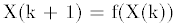(1)

where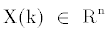is the state vector of the drive system and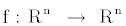. As the response system we consider the following chaotic system described by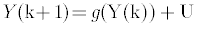(2)

Where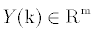the state vector of the response system is,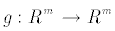is the nonlinear part of the response system and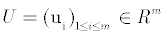is the vector controller.

Definition 1: The drive system (1) and the rsponse system (2) are said to be synchronized in dimension d, with respect to scaling matrice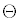and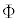, respectively, if there exists a controllerand a given matrice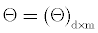and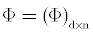such that thesynchronization error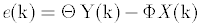(3)

Satisfies that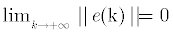Because in real world all chaotic maps are described in 2D and 3D, we restrict our study about double scaling matrice synchronization to 2D chaotic maps and 3D discrete hyperchaotic systems this restriction does not lose the generality of our main results.

Synchronization of 2D Drive System and 3D Response System in 2D

In this section, the drive and the response chaotic systems are in the following forms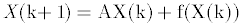(4)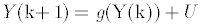(5)

Where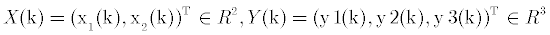are state vectors of the drive system and the response system, respectively,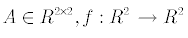is the nonlinear part of the drive system (4),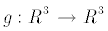and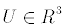is the vector controller.

The synchronization error between the drive system (4) and theresponse system (5) can be derived as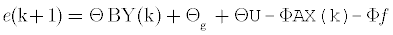(6)

Where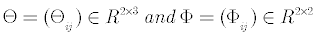are scaling matrices. To achieve synchronization between systems (4) and (5), we assume that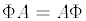(7)

And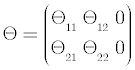(8)

The error system between the drive system (4) and the response system (5), can be written as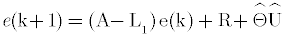(9)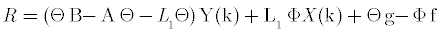(10)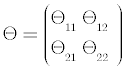(11)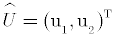(12)

And L is 2x2 control matrix to be determined. The controller is chosen as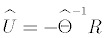(13)

Where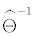is the inverse matrix of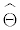By substituting Equation (13) in Equation (9), the error system can be written as.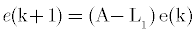(14)

Theorem 2: If there exists a positive definite matrix P, such that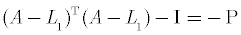(15)

Then, the drive system (4) and the response system (5) are globally synchronized, with respect to scaling matriceand,. under the controller. (13).

Proof: Construct the candidate Lyapunov function in the form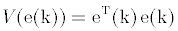(16)

We obtain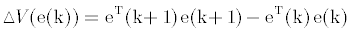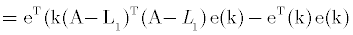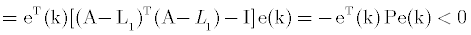Thus, from the Lyapunov stability theory, it is immediate that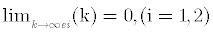: That is the zero solution of the error system (14) is globally asymptotically stable and therefore, systems (4) and (5) are globally synchronized.

#### Synchronization of 2D Drive System and 3D Response System in 3D

In this section, the drive and the response chaotic systems are in the following forms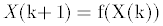(17)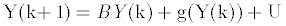(18)

Where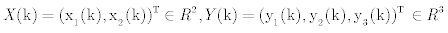state vectors of the drive system and the response system are, respectively,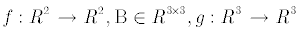is nonlinear part response system andis the vector controller. To achieve synchronization between systems (17) and (18), we assume that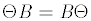(19)

Then, the error system between the drive system (17) and the response system (18), can be derived as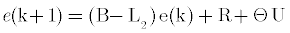(20)

Where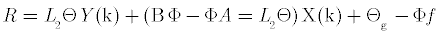(21)

And L2 is 3x3 control matrix to be determined. We choose the controller U as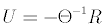(22)

Where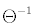is the inverse matrix ofTheorem 3 If L2 is chosen such that all eigenvalues of B-L2 are strictly inside the unit disk, then the drive system (17) and the response system (18) are globally synchronized with respect toandunder the control law (22).

Proof: By substituting Equation (22) in Equation (20), the error system can be written as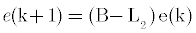(23)

Thus, by asymptotic stability of linear discrete-time systems, if all eigenvalues of B-L2 are strictly inside the unit disk, it is immediate that all solution of error system (23) go to zero as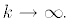Therefore, systems (8) and (9) are globally synchronized.

#### Synchronization of 2D Drive System and 3D Response System in 4D

In this section, the drive and the response chaotic systems are in the following forms(24)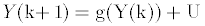(25)

Where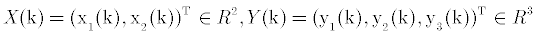state vectors of the drive system and the response system, respectively,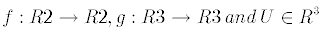is the vector controller.

The error system between the drive system (24) and the response system (25) can be derived as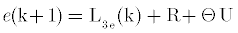(26)

Where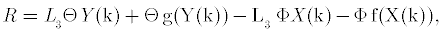(27)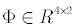is arbitrary scaling matrix,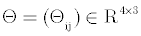is scaling matrix described as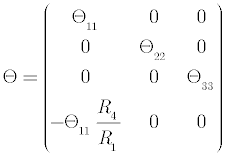(28)

Where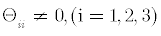and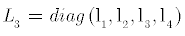is control matrix. Then, the error system between systems (24) and (25), can be written as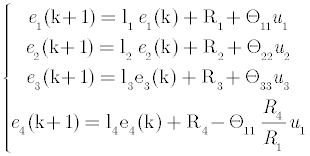(29)

To achieve synchronization, we choose the controller U as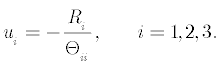(30)

By substituting Equation (30) in Equation (26), the error system can be written as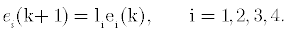(31)

Theorem 4: If L3 is chosen such that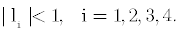(32)

Then, the drive system (24) and the response system (25) are globally synchronized, with respect toand, under the control law (30).

Proof: We take as a candidate Lyapunov function: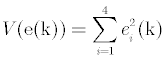(33)

We get: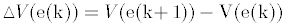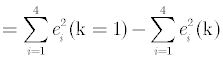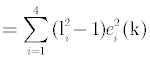By using (32), we obtain: ΔV(e(k)) < 0 Thus, by Lyapunov stability it is immediate that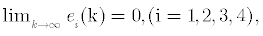and from the fact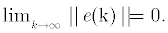We conclude that the systems (24) and (25) are globally synchronized.

#### Numerical Application

Now, we consider 2D Hénon map as the drive system and the controlled 3D hyperchaotic Baier-Klein map as the response system. The Hénon map is can be described as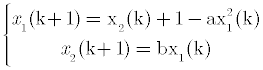(34)

Which has a chaotic attractor, for example, when (α,β) = (1.4, 0.2)  . The Henon map chaotic attractor is shown in Figure 1.

The controlled the Baier-Klein map can be described as :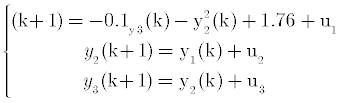(35)

Where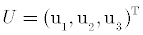is the vector controller. The chaotic attractors of Baier Klein map is shown in Figure 2.

Case 1: Synchronization of Hénon map and Baier-Klein map in 2D

According to our approach presented in section 3, we obtain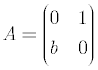(36)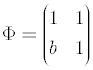(37)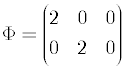(38)

and the control matrix L as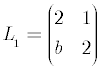(39)

Using simple calculations, we can show that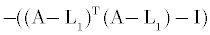is a positive de…nite matrix. Therefore, in this case, systems (34) and (35) are synchronized in 2D. The error functions evolution is shown in Figure 3.

Figure 3: Time evolution of synchronization errors: e1 and e2 between 2D Henon map and 3D hyperchaoticBaier-Klein map in 2D.

Case 2: Synchronization of Hénon map and Baier-Klein map in 3D

According to our approach presented in section 4, we obtain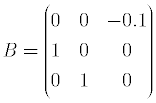(40)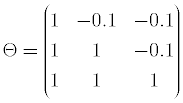(41)

And the control matrix L2 as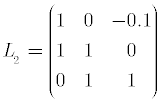(42)

Simply, we can show that all eigenvalues of B - L2 are strictly inside the unit disk. Therefore, in this case, systems (34) and (35) are synchronized. The error functions evolution is shown in Figure 4.

Figure 4: Time evolution of synchronization errors e1, e2 and e3 between 2D Henon map and 3D hyperchaotic Baier-Klein map in 3D.

Case 3: Synchronization of Hénon map and Baier-Klein map in 4D

According to our approach presented in section 5, we obtain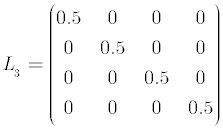(43)

Finally, it is easy to know that the conditions of Theorem 3 are satisfied. Therefore, in this case, systems (34) and (35) are synchronized. The error functions evolution is shown in Figure 5.

Figure 5: Time evolution of synchronization errors e1, e2, e3 and e4 between 2D Henon map and 3D hyperchaotic Baier-Klein map in 4D.

#### Conclusion

In this paper, a new type of synchronization with double scaling matrice was proposed and new synchronization result are derived using new control schemes and Lyapunov stability theory. Firstly, when the dimension of synchronization is the same of the response system the synchronization control is achieved by controlling the linear part of the response system. Secondly, if synchronization is made in dimension of the drive system, the linear part of the drive system is controlled. Finally, synchronization is guaranteed by new diagonal matrix when the synchronization dimension is greater than the dimensions of drive and response systems. Numerical example and simulations results were used to verify the effectiveness of the proposed schemes.

#### References

Select your language of interest to view the total content in your interested language

### Article Usage

• Total views: 12008
• [From(publication date):
December-2014 - Aug 21, 2019]
• Breakdown by view type
• HTML page views : 8212
• PDF downloads : 3796

## Post your commentCan't read the image? click here to refresh
###### Peer Reviewed Journals

Make the best use of Scientific Research and information from our 700 + peer reviewed, Open Access Journals

International Conferences 2019-20

Meet Inspiring Speakers and Experts at our 3000+ Global Annual Meetings

Top